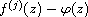Electron. J. Diff. Equ., Vol. 2012 (2012), No. 226, pp. 1-15.

### Growth of solutions to linear differential equations with entire coefficients Hui Hu, Xiu-Min Zheng

Abstract:
In this article, we study the growth of solutions of linear differential equations with some dominant entire coefficients. Especially, we obtain some results on the iterated p-lower order of these solutions, which extend previous results. Moreover, we investigate the iterated exponent of convergence of distinct zeros of.

Submitted May 8, 2012. Published December 17, 2012.
Math Subject Classifications: 30D35, 34M05.
Key Words: Iterated p-order; iterated p-lower order; iterated p-lower type; iterated exponent of convergence of distinct zeros.

Show me the PDF file (283 KB), TEX file, and other files for this article.Hui Hu Institute of Mathematics and Information Science Jiangxi Normal University, 330022, China email: h_h87_6@hotmail.com Xiu-Min Zheng Institute of Mathematics and Information Science Jiangxi Normal University, 330022, China email: zhengxiumin2008@sina.com